# Numb3rs 220: Guns and Roses

Alas, there's little actual mathematics in this episode, so we'll explore the next best thing: some physics. In particular, we'll look at the Doppler effect, what it is, when it occurs, and how we can use it.

## The Frequencies they are A-changin'

You've probably noticed the Doppler effect many times before. It's the change of a siren's pitch you hear as an ambulance or a firetruck passes by you. To understand why in such situations we hear the pitch change we must first learn a little about waves.
A light wave (or electromagnetic wave, as it's properly called) differs from other types of waves in that it does not require a medium in which to propagate: electromagnetic waves travel through empty space, while for example sounds waves can only travel through a gas, liquid, or a solid. Another, related peculiarity of electromagnetic waves is that they act both like waves and like particles! This puzzling phenomenon has motivated much of the development of modern physics and the discovery that all particles have wave-like properties.

A traveling wave, whether occurring in water (e.g. sea wave), air (sound wave), or vacuum (light wave), looks something like this in action (courtesy of wikipedia). You can see a snapshot representation of a wave to the left below. The distance between two consecutive peaks or troughs is called the wavelength is usually represented by the Greek letter lambda (λ). The time it takes for a wave to travel one wavelength, the distance λ, is called a period, and we'll denote it by T. In the picture to the right below, a source is producing a continuous wave of a given wavelength. The circles represent the peaks of the wave, the distance between them equaling the wavelength.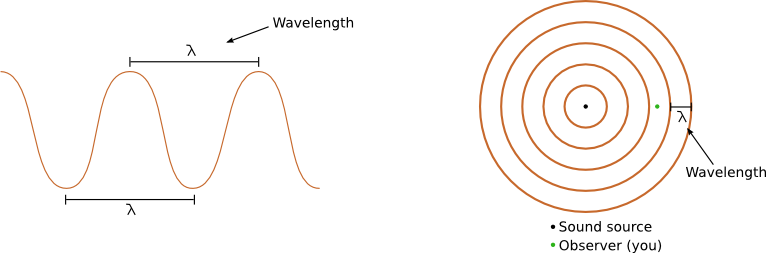Activity 1:
1. What is the relation between a wave's speed, wavelength, and period? (Hint: it's not hard, try. If you're stuck, look here.)
2. The quantity 1/T is called the frequency of the wave. It corresponds to the pitch we hear when a sound wave hits our eardrums and the color we see when a light wave hits the retina. Rewrite the above relation in terms of frequency instead of period.

Suppose now that the source of the wave is moving toward you, as in the picture in activity 2 below. While the source is still emitting a fixed frequency wave, the perceived wavelength now depends on where the observer is located with respect to the source.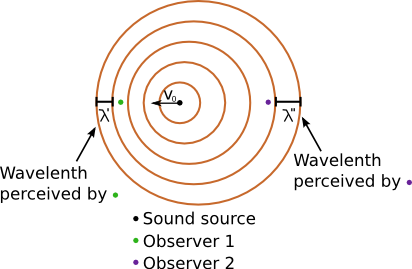Activity 2:
1. Assume the source is moving toward you, the observer, with speed v0, and the wave emitted is a sound wave of frequency f. Then the corresponding wavelength λ, by activity 1 above, is equal to vs/f, where vs is the speed of sound in air (around 345 meters/second). What does the perceived wavelength λ' equal to in terms of v0, T, and λ?
2. What does λ' equal to in terms of the speed of sound vs and the perceived frequency, which we'll denote f’? (Hint: use activity 1.)
3. Write the equation from part 1 above in terms of f, f’, vs and v0.

If you did the algebra correctly, you should have gotten something equivalent tofor #3 above. What this tells us is that perceived sound frequency, the pitch, increases relative to the original frequency as v0 increases.

Activity 3:
1. Suppose that instead of moving toward you, the source of the sound moves away from you with speed v0. How does the equation for the perceived frequency f’ change?
2. What happens if the sound source is stationary, but you, the observer, are moving toward it with speed v0? Draw a picture, work it out!
3. What if the sound source approaches you with a speed greater than vs? I.e. what if v0 ≥ vs?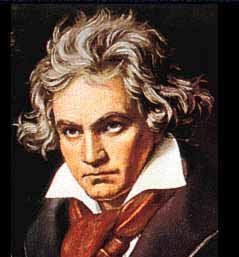Activity 4:
1. Suppose you're standing by a sound source which emits a a single frequency tone for a split second. How fast will you need to run away from the sound source to hear the tone for as long as you keep running? (Ignore the issue of volume, which corresponds, by the way, to a wave's amplitude or height.)
2. The orchestra has just finished playing Beethoven's 5th symphony, and you, a member of the audience, suddenly feel a strong urge to hear it again, but backwards! How fast would you have to run away from the concert hall to hear it replayed from finish to start at the pitch it was originally performed?

As you may have noticed, we have so far ducked the original question! All of the above discussion does not explain why we hear the pitch of a siren change as it passes us. The reason is that we, the observer, are not in the path of the sound source as that happens: the ambulance passes by us, not through us (one may hope). Thus while the pitch did not change for the observers in the diagram in activity 2, it would if the observers were not on the line of motion, as in the illustration to the right. The key to describing how the perceived frequency changes is to note that we only care about the speed of the source relative to the observer. Thus the only modification needed is to figure out what this speed is in the case where the observer is not on the line of motion (see illustration below).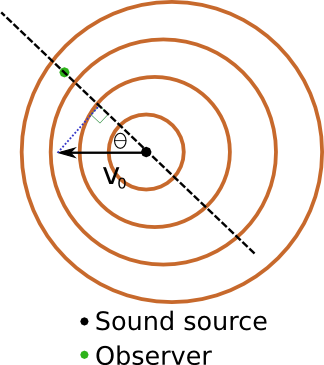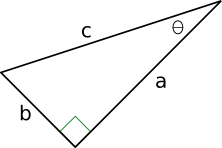Activity 5:
1. Recall a bit of trigonometry: in a right triangle to the right, what is length of side a in terms of angle θ and the hypotenuse c?
2. As in the picture above, the sound source is moving West with speed v0. What is the speed of the source relative to the observer at an angle θ North of it? (Hint: use part one and see illustration above.)